# Convert Word Document to Excel

This post will guide you how to convert or transfer a word document to an excel worksheet in excel. How do I transfer data from word forms to an Excel worksheet.  How to import-convert data from word document into excel worksheet.

Convert Word Document to Excel

If you want to convert a word document to an excel worksheet, you should save your word document as type to a plain-text file, then importing and exporting plain-text file from Microsoft excel. And Excel can open plain-text file to convert the content into a worksheet. So just do the following steps:

#1 open your word document that you want to convert to an excel worksheet. Then go to File tab, click Save As… button from the drop-down menu list.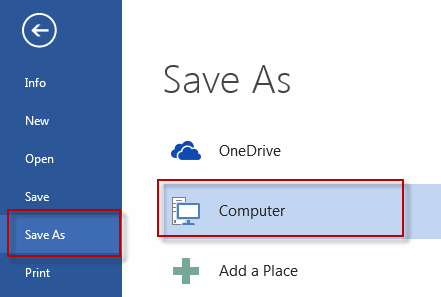#2 choose “Plain Text” from the drop-down list of Save as type: list box. Click Save button. Then a copy of your word document is saved to your compute as a .TXT file.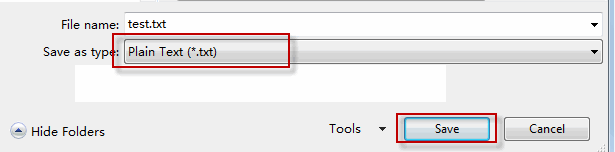#3 open Excel, then go to Data Tab, click From Text command under Get External Data group.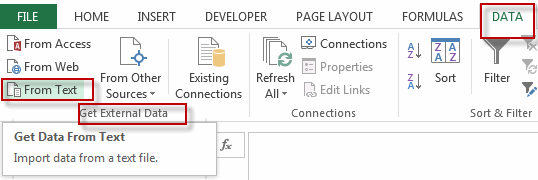#4 select your word document that is converted to plain text, then click Import button.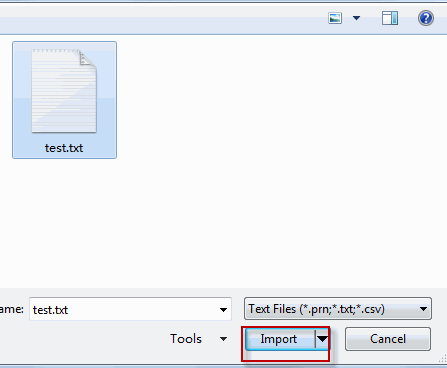#5 select Delimited option in the Text Import Wizard, click Next button.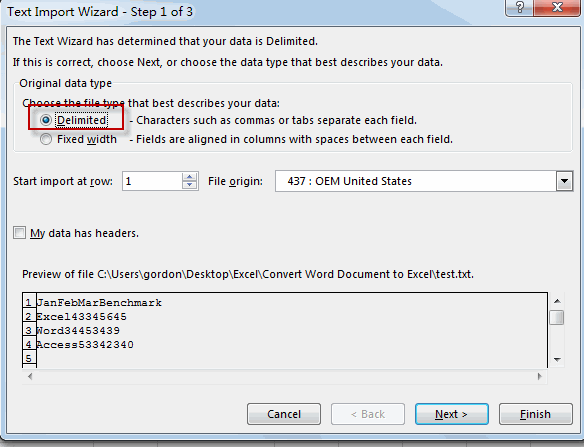#6 Select Delimiters from the Delimiters section. Such as: Tab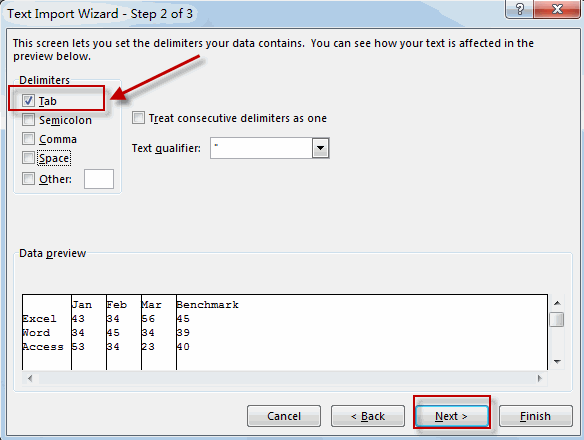#7 click Finish button.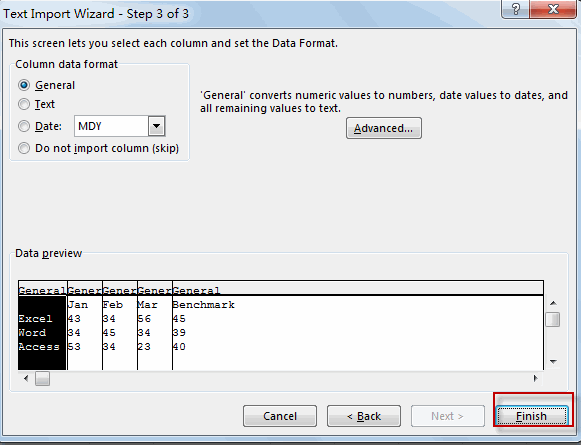#8 select one cell that you want to put the data in. then click OK button.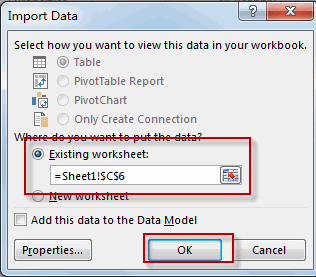#9 let’s see the last result.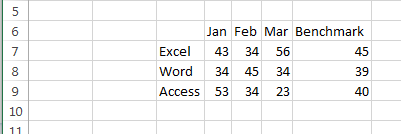Related Posts

Cap percentage values between 0 and 100

This article will talk about how to cap the percentage values between 0% and 100% in Microsoft Excel Spreadsheet or Google Sheets. If you are a newbie on Excel or google Sheets, you may be able to do this by ...

Calculate Cap Percentages to Specific Value

This article will talk about how to limit the cap percentage of a given amount to a given value in Microsoft Excel Spreadsheet or Google Sheets. If you are a newbie on Excel or google Sheets, you may be able ...

Calculate Win Loss Tie

Suppose you got a task to calculate the win, loss, and tie totals; what would you do? If you are new to Ms Excel and don't have enough experience with it, then you might do this task manually but let ...

Calculate Years Between Dates In Ms Excel

If you are an avid Ms Excel user, then you might have come across a task in which you needed to calculate the years between the dates; you might take it easy and do this task manually, which is also ...

Calculate Number of Hours between Two Times

Calculating the difference between two times might be a valuable statistic for subsequent computations or averages, whether you're producing a time sheet for staff or recording personal exercises. While Excel has a plethora of complex functions, including date and time ...

Calculate Loan Interest in Given Year

When you borrow money, you are supposed to repay it gradually. Lenders, on the other hand, want to be compensated for their services and the risk they incur by lending you money. That is, you will not just repay the ...

Calculate Interest Rate for Loan

The interest rate is the fee charged by a lender to a borrower and is expressed as a percentage of the principal—the lent amount. The interest rate on a loan is often expressed as an annual percentage rate, abbreviated as ...

Calculate Interest for Given Period

Using the IPMT function in Excel, we can compute the interest payment on any loan. This step-by-step tutorial will guide Excel users of all skill levels through the process to calculate interest for given period. Finally, the formula: =IPMT(B3/12,1,B5,-B2) The ...

How To Use Excel GCD Function

This post will guide you how to use Excel GCD function with syntax and examples in Microsoft excel. Description The Excel GCD function Returns the greatest common divisor of two or more integers. So you can use the GCD function ...

Calculate A Ratio From Two Numbers In Excel

In elementary mathematics, a ratio is a connection or comparison between two or more integers. For example, ratios are often expressed as ":" to demonstrate the relationship between two numbers. You would think that manually calculating a ratio from two ...

Sidebar